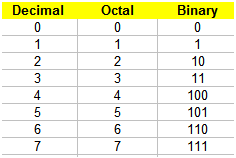# Octal to decimal - Two methods in Matlab

Basics

Video

Idea 1

Idea 2

### Basics

To convert a value from octal to decimal (base 8 to base 10), we first need to know what an octal number is.

The octal system is a numerical system used in digital electronics. In this system of numbers, the symbols are counted only from ‘0’ to ‘7’. In base 8 we only have eight different symbols (there are no symbols such as '8' or '9').

Similarly, the decimal system (base 10) has ten symbols, '0' to '9' and the binary system (base 2) has only two symbols, '0' and '1'.

The following table shows the meaning of all the symbols in the octal system and the equivalents in the decimal and binary systems.

Table equivalents: decimal, octal and binary numbersYou can see that from 0 to 7 a number in octal is exactly the same as it is in decimal, but what if you need to go beyond 7?

### Video

Here's a video that explains you the concepts behind the numerical equivalencies in different systems. After the video, we show you how to code that in Matlab.

### Solution 1. Conversion from Octal to Decimal using the obvious way in Matlab

We can go from octal to decimal by using the function base2dec, which converts a base-N number string to a decimal number. Its syntax is

base2dec('string', base)

The parameter base can be anything, not only 8.

For example, base2dec('100', 8) produces a 64 in decimal and
base2dec(
'1352', 8) produces a 746.

It’s important to remember that octal numbers are treated as strings.

### Solution 2. Conversion using a different approach

This is the idea that is shown on the video.

clear, clc,  format compact

% Here we keep our octal number
o = '317'

% The decimal equivalent starts being 0
d = 0;
% Let's flip the octal symbol to align with the
% exponents that follow

f = fliplr(o);
% Now we create the appropriate exponent
for p = 0 : length(o) - 1

% This is the necessary multiplication and addition
% to achieve our idea, digit-by-digit
d = d + str2num(f(p+1)) * 8^p;
end
% Now, we just display the result
d

If you run the code above, you'll get this result on screen (edited for clarity).From 'Octal to decimal' to home

From 'Octal to decimal' to 'Matlab Programming'